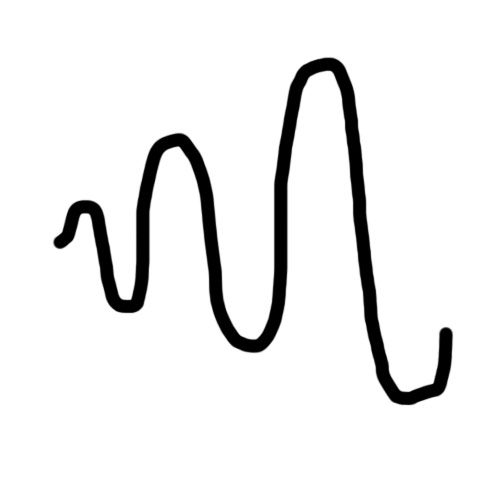# Weapon Bullet Question - Steadily increasing sine wave

So I have a gun for a game I’m working on where the bullets travel in a sine wave, however the idea I want to implement is to have the bullets y-value smoothly increase the size of the sine wave in a way that’s not noticeably jagged. So the sine wave starts small and then increases reaching a maximum point.

The following code is here:

``````using System.Collections;
using System.Collections.Generic;
using UnityEngine;

public class BulletMove : MonoBehaviour {

public Vector3 startPosition;
public Vector3 endPosition;
public Vector3 bend;
public float bendRatio;
public float amp;

// Use this for initialization
void Start () {
bendRatio = 1;
amp = 5;
startPosition = transform.position;
endPosition = new Vector3 (5, 0, 0);
bend = new Vector3 (0, bendRatio, 0);
}

// Update is called once per frame
void Update () {

Vector3 currentPosition = Vector3.Lerp (startPosition, endPosition, Time.time);

currentPosition.y += bendRatio* Mathf.Sin((Time.time * amp) * Mathf.PI);

transform.position = currentPosition;

}
}
``````

I tried doing this in a new Unity file just to see if I could get the sine wave to increase but after trying numerous things I wasn’t able to get it to do what I want. I’m not sure when to update my bendRatio variable to increase the size of the sine wave. I can’t do it every frame, not even by 1 or the sine wave gets out of control. I’d also like if possible to hit predetermined heights just in case the sine wave gets out of control. So bendRatio would start at 1, then go to 1.5 and then 2 etc, but when that would be updated I don’t know.

Ideally, I would like the wave to create this shape.Please let me know if this is even possible.

Of course it’s possible!

A few things first though. To make things a little easier, I would use transform.Translate over Vector3.Lerp. This is because Vector3.Lerp isn’t a consistent speed and as such would make it a little more difficult to sync things up.

Next, on line 27 you have Mathf.PI outside the sin function. If you were trying to convert to meters, it should look something like this instead:

``````Mathf.Sin (x * 2 * Mathf.PI)
``````

This is because Mathf.Sin takes radians, and there are 2pi radians in a circle. So to convert to meters you have to cancel the radians by multiplying.

Okay, so here’s some code:

``````    public float amplitude = 2f;
public float amplitudePlus = 0.5f;
public float speed = 2;
public float cyclesPerSecond = 1;

float curTime = 0;

void Update () {
transform.Translate (transform.forward * speed * Time.deltaTime);

transform.position = new Vector3 (amplitude * Mathf.Sin (cyclesPerSecond * curTime * 2 * Mathf.PI), transform.position.y, transform.position.z);

amplitude += amplitudePlus * Time.deltaTime;
curTime += Time.deltaTime;
}
``````

I’m gonna do my best to explain this, hopefully I succeed. We have 4 variables, amplitude, amplitudePlus, speed, and cyclesPerSecond. Amplitude will be the starting amplitude of our function, and we will add to it with amplitudePlus. Speed will be how fast the projectile is, and cyclesPerSecond will be how fast the projectile oscillates back and forth per second. The fifth variable, curTime, will keep track of our time to be used in our sin function.

The first line in Update () is pretty self explanatory. We are moving the projectile forward by our speed. Time.deltaTime is used here to make sure that we move in meters per second, and not meters per frame.

The next line is where the sin function is located. Inside Mathf.Sin there is the cyclesPerSecond times curTime times 2 times Mathf.PI. curTime is where on the sin wave we are. cyclesPerSecond scales the sin function on the x-axis to increase the cycles per period. Next it is multiplied by 2 times Mathf.PI to cancel the unit radians. So now, the period of the function is in seconds, and it will oscillate cyclesPerSecond time per second. This is because on the last line we are adding seconds to curTime:

``````curTime += Time.deltaTime;
``````

Time.deltaTime being the time between each frame. Finally, the last thing that is done is the entire sin function is multiplied by the amplitude, scaling the function on the y-axis.

The second to last line is where amplitude is increased. Here I have it setup in terms of time as well. So the amplitude of the function will increase by amplitudePlus amount per second.

Time.deltaTime is important when looking at rates of change in Unity. Time.deltaTime is the approximate time between frames. You can use this value to ensure that you add values in terms of seconds, not frames.

Hope this was helpful, let me know if something needs clarified.